Fibres of Orientations edit page

A fibre in orientation space is essentially a line connecting two orientations and can be represented in MTEX by a single variable of type fibre. To illustrate the definition of a fibre we first define cube and goss orientation

and then the fibre connecting both orientations

Finally we plot everything into the Euler space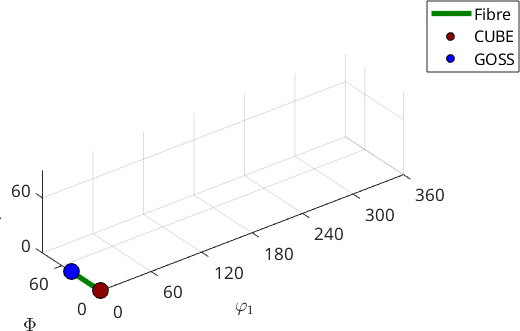Alternatively, we may visualize the fibre also in axis angle space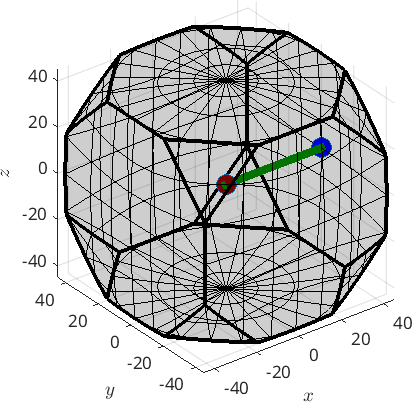Obviously, f is not a full fibre. Since, the orientation space has no boundary a full fibre is best thought of as a circle that passes trough two fixed orientations. In order to define the full fibre us the option 'full'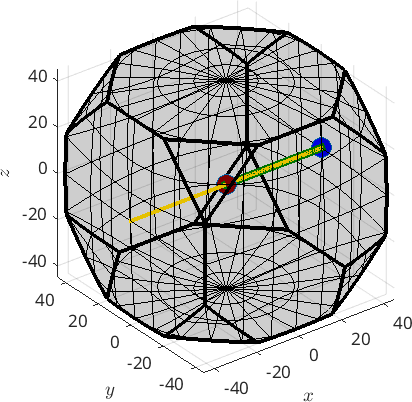## Fibres in pole figures and inverse pole figures

MTEX supports for fibres all the plotting options that are available for orientations. This included pole figures and inverse pole figures using the commands plotPDF and plotIPDF.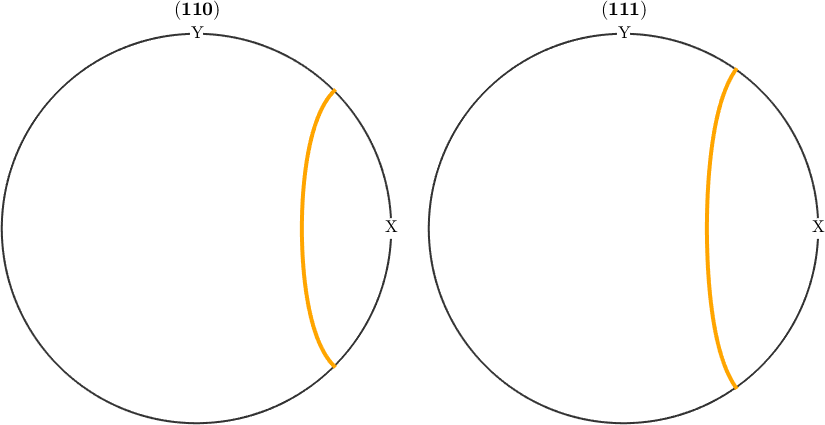An important difference to orientation plots is that fibres are not automatically symmetrised when plotted. To achieve this use the command symmetrise.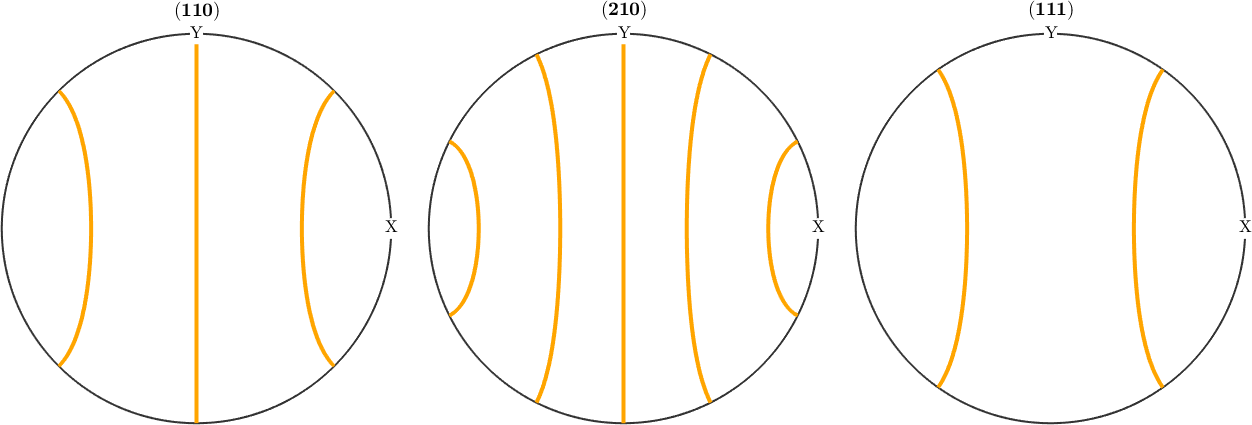Inverse pole figures are by default restricted to the fundamental sector. You may use the option 'complete' to plot the entire sphere.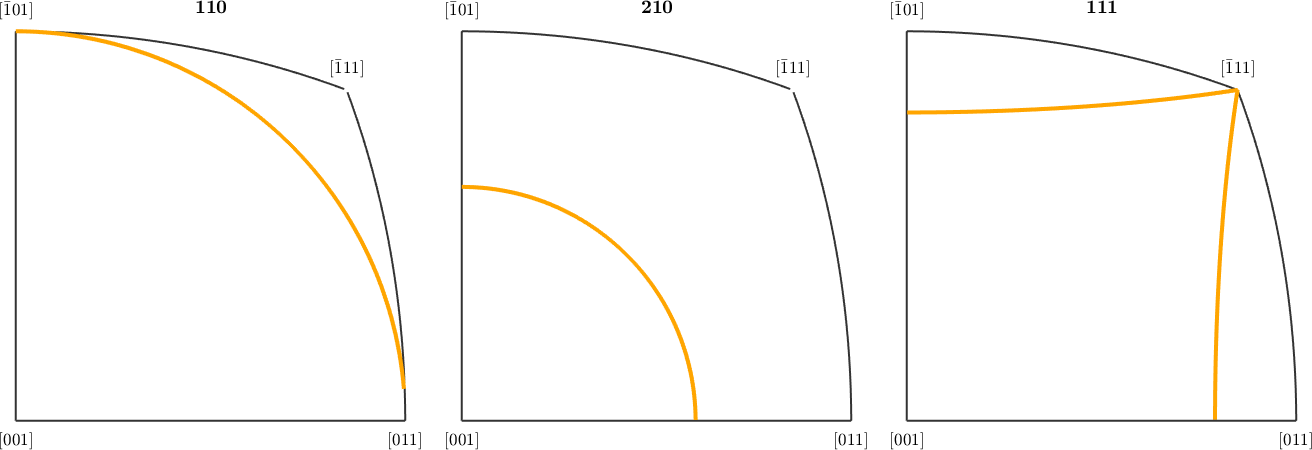## Defining a fibre by directions

Alternatively, a fibre can also be defined by a pair of a crystal and a specimen direction. In this case it consists of all orientations that alignes the crystal direction parallel to the specimen direction. As an example we define the fibre of all orientations such that the c-axis (001) is parallel to the z-axis by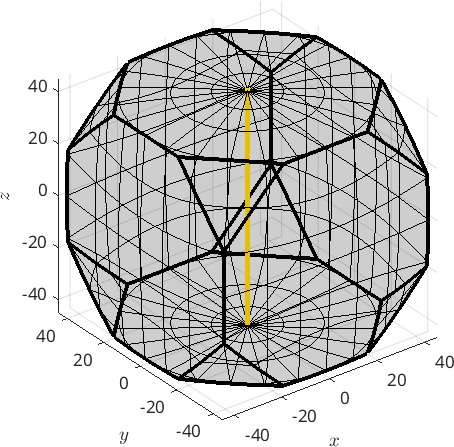If both directions of type Miller the fibre corresponds to all misorientations which have these two direcetion parallel.

Finally, a fibre can be defined by an initial orientation ori1 and a direction h, i.e., all orientations ori of this fibre satisfy

The following code defines a fibre that passes through the cube orientation and rotates about the (111) axis.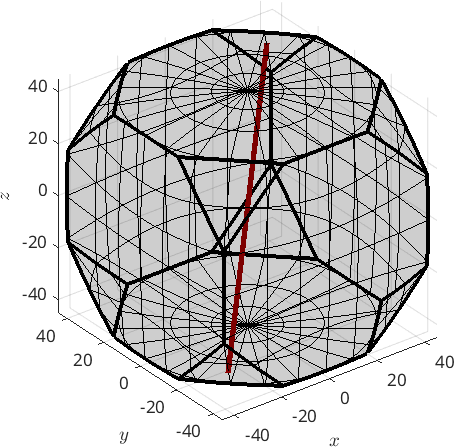## Predefined fibres

MTEX includes also a list of predefined fibres, e.g., alpha, beta, gamma, epsilon, eta, tau and theta fibres. Those can be defined by

Lets plot an overview of all predefined fibres with respect to orthorhombic specimen symmetry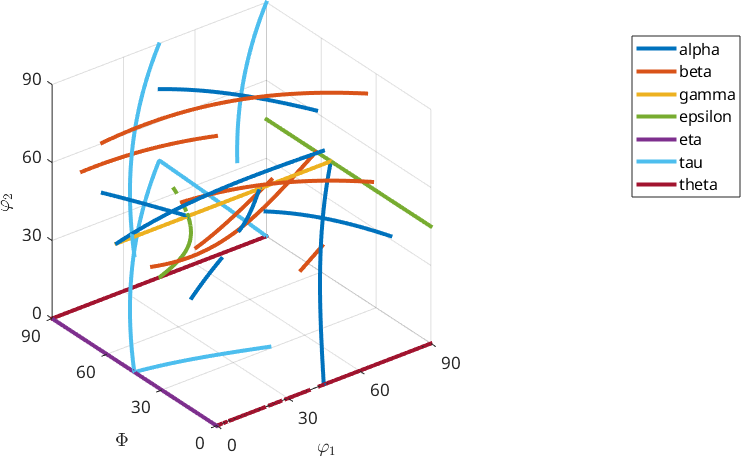## Fibre ODFs

Note, that it is straight forward to define a corresponding fibre ODF by the command fibreODF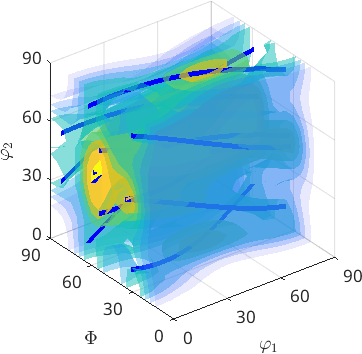## Visualize an ODF along a fibre

We may also visualize an ODF along a fibre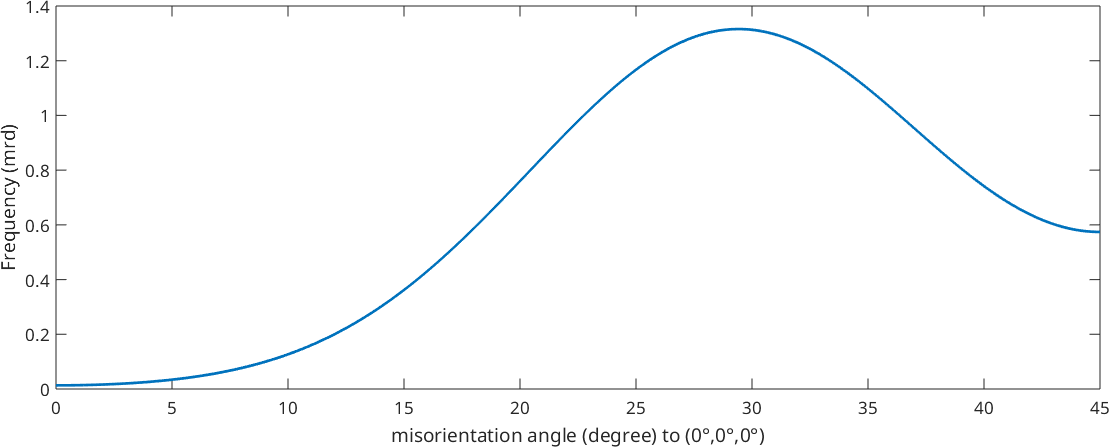## Compute volume of fibre portions

or compute the volume of an ODF in a tube around a fibre using the command volume